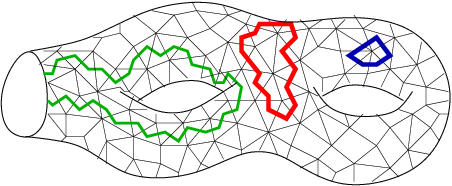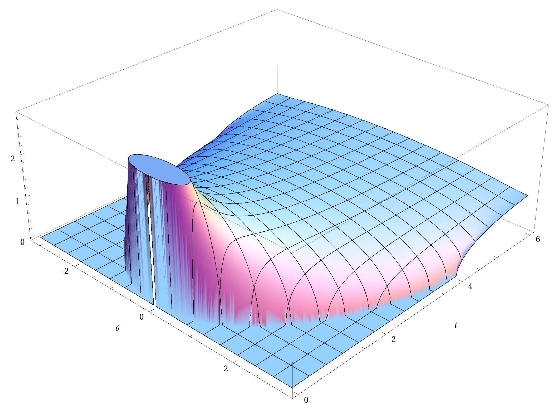# GranMa 2011

## Institut Henri Poincaré, Paris

General

Announcement

Participants

Practical details

Registration

Schedule

Alain Rouault, UVSQ (Versailles):

## Truncations of Haar distributed matrices, traces and bivariate Brownian bridges

(joint work with Catherine Donati-Martin) Let $U$ be a Haar distributed matrix in $\mathbb U(n)$ or $\mathbb O(n)$. We show that after centering the two-parameter process $W^{(n)} (s,t) = \sum_{i \leq \lfloor ns \rfloor, j \leq \lfloor nt\rfloor} |U_{ij}|^2$ converges in distribution to the bivariate tied-down Brownian bridge.